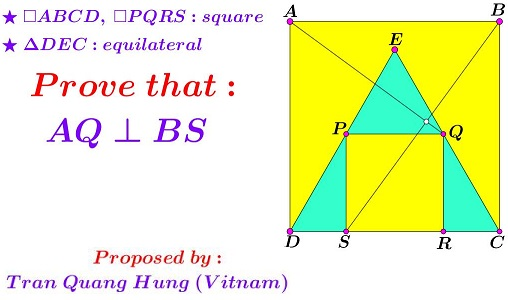# Equilateral triangle and squares

### Source### Problem### Solution 1

Assume $AB=1$ and denote $SR=x.$ Let $H$ be the intersection of $AD$ and $PQ.$We first show that two right triangles $AHQ$ and $SCB$ are similar. Indeed, in $\Delta DPS,$ the Pythagorean theorem gives $DS^2+PS^2=DP^2,$ i.e., $\displaystyle \left(\frac{1-x}{2}\right)^2+x^2=\left(\frac{2x}{\sqrt{3}}\right)^2$ from which $x=2\sqrt{3})-3.$

To continue, $\displaystyle CS=x+\frac{1-x}{2}=\frac{1+x}{2}$ and similarly $\displaystyle HQ=\frac{1+x}{2}.$ On the other hand, $AH=1-x.$ It is now easy to check that $\displaystyle \frac{AH}{HQ}=\frac{PS}{CS},$ making triangles $AHQ$ and $SCB$ similar. Two pairs of their corresponding sides are perpendicular and so are the remaining pair: $AQ\perp BS.$

Consider $G\in BC$ with $\displaystyle CG=\frac{x}{2}.$ By simple calculations, $G$ is equidistant from $B,$ $P$ and $S.$ Thus, if $GF\parallel CD,$ with $F\in (BPS),$ then $\stackrel{\frown}{PF}=\stackrel{\frown}{FS}.$ Since also $GF=GB,$ $F\in BD,$ proving $\angle PBD=\angle PBF =\angle DBS.$

### Solution 2

WLOG, let $AB=1$. Let $SR=p$. Thus, in $\Delta QCR$,

$\displaystyle \tan~60^o=\sqrt{3}=\frac{QR}{RC}=\frac{2p}{1-p}\Rightarrow p=2\sqrt{3}-3.$

Choosing $X$ and $Y$ axes along $BA$ and $BC$,

\displaystyle \begin{align} \vec{AQ}\cdot\vec{BS}&=\left[\left(\frac{1-p}{2}-1\right)\hat{x}+(1-p)\hat{y}\right]\cdot\left[\left(\frac{1-p}{2}+p\right)\hat{x}+\hat{y}\right] \\ &=\left(-\frac{1+p}{2}\hat{x}+(1-p)\hat{y}\right)\cdot\left(\frac{1+p}{2}\hat{x}+\hat{y}\right)=(1-p)-\frac{(1+p)^2}{4} \\ &=\left(4-2\sqrt{3}\right)-\left(\sqrt{3}-1\right)^2=0. \end{align}

Thus, $AQ\perp BS$. Let the extension of $BP$ intersect $AD$ at $Z$ and let $\vec{BZ}=\hat{x}+k\hat{y}.$

$\displaystyle \vec{BP}=\left(\frac{1-p}{2}+p\right)\hat{x}+(1-p)\hat{y}=\frac{1+p}{2}\hat{x}+(1-p)\hat{y}.$

Equating the ratios of the $X$ and $Y$ components of $\vec{BP}$ and $\vec{BZ},$

$\displaystyle k=AZ=\frac{2(1-p)}{1+p}=\sqrt{3}-1.$

Also,

$\displaystyle CS=\frac{1-p}{2}+p=\sqrt{3}-1.$

Thus, $AZ=CS$ and by symmetry $\angle ZBD~(=\angle PBD)=\angle DBS$.

### Solution 3

1. We center the coordinates at D. The product of the slopes of AQ and BS is

$\displaystyle \frac{AD-QR}{-RD}\frac{BC}{SC}.$

If we denote $SD=x,SR=y$, the above product is

$\displaystyle \frac{2x(2x+y)}{-(x+y)^2}=-1$

using that $y=\tan(60)=\sqrt{3}x$

2. By 1., the rotation counterclockwise at 90 degrees of $BS$ is $AQ$. Also, the rotation at 90 degrees of $BD$ is $AC$. Hence the angle between $BS$ and $BD$ is the one between $AQ$ and $AC$ which is by symmetry same as between $BP$ and $BD$.

Inspiration for 2. D. Schleicher, M.Lackmann, An invitation to mathematics, p.26

### Acknowledgment

The problem that was originally posted at the Peru Geometrico facebook group by Miguel Ochoa Sanchez was kindly brought to my attention by Stan Fulger. Solution 2 is by Amit Itagi; Solution 3 is by Bogdan Lataianu.